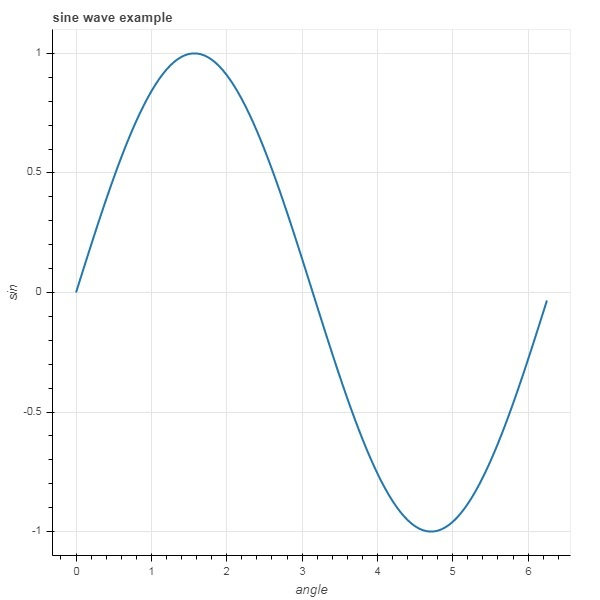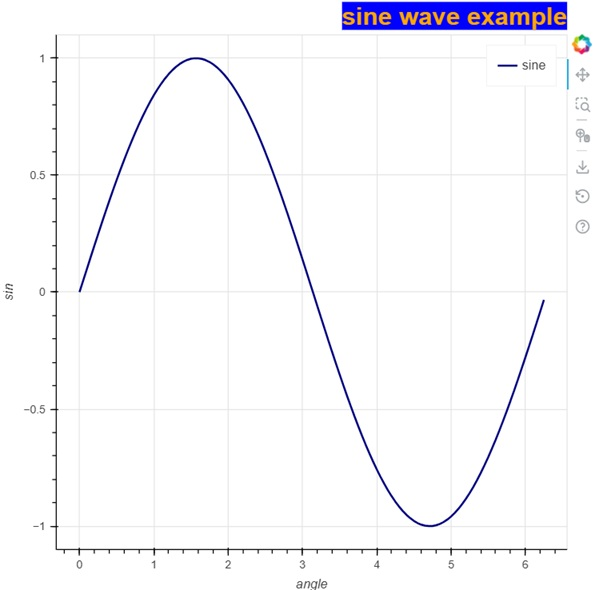# Bokeh - Annotations and Legends

Annotations are pieces of explanatory text added to the diagram. Bokeh plot can be annotated by way of specifying plot title, labels for x and y axes as well as inserting text labels anywhere in the plot area.

Plot title as well as x and y axis labels can be provided in the Figure constructor itself.

```fig = figure(title, x_axis_label, y_axis_label)
```

In the following plot, these properties are set as shown below −

```from bokeh.plotting import figure, output_file, show
import numpy as np
import math
x = np.arange(0, math.pi*2, 0.05)
y = np.sin(x)
fig = figure(title = "sine wave example", x_axis_label = 'angle', y_axis_label = 'sin')
fig.line(x, y,line_width = 2)
show(p)
```

### OutputThe title’s text and axis labels can also be specified by assigning appropriate string values to corresponding properties of figure object.

```fig.title.text = "sine wave example"
fig.xaxis.axis_label = 'angle'
fig.yaxis.axis_label = 'sin'
```

It is also possible to specify location, alignment, font and color of title.

```fig.title.align = "right"
fig.title.text_color = "orange"
fig.title.text_font_size = "25px"
fig.title.background_fill_color = "blue"
```

Adding legends to the plot figure is very easy. We have to use legend property of any glyph method.

Below we have three glyph curves in the plot with three different legends −

```from bokeh.plotting import figure, output_file, show
import numpy as np
import math
x = np.arange(0, math.pi*2, 0.05)
fig = figure()
fig.line(x, np.sin(x),line_width = 2, line_color = 'navy', legend = 'sine')
fig.circle(x,np.cos(x), line_width = 2, line_color = 'orange', legend = 'cosine')
fig.square(x,-np.sin(x),line_width = 2, line_color = 'grey', legend = '-sine')
show(fig)
```

### Output